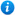# Adding and Subtracting Fractions - Year 3

Adding and Subtracting Fractions - Year 3

Year 3 maths programme of study - Number - fractions

• add and subtract fractions with the same denominator within one whole
'Adding and Subtracting Fractions - Year 3' is a KS2 maths teaching resource ideal to use in a lesson covering the year 3 curriculum objective listed above. This colourful and engaging PowerPoint presentation can be used to introduce pupils to fraction addition and subtraction when the denominators are the same, or as a review of the topic. Content includes:

• What are fractions recap
• How to add fractions with the same denominator explanation
• Adding fractions activity with a differentiated worksheet
• How to subtract fractions with the same denominator explanation
• Subtracting fractions activity with a differentiated worksheet
• Adding and subtracting fractions word problems with accompanying worksheet
• 1 further differentiated worksheet
• 1 further worksheet

'Adding and Subtracting Fractions - Year 3' can be edited so teachers are able to adapt the resource to meet the needs of each class they teach.

'Adding and Subtracting Fractions - Year 3' can be previewed in full by clicking on the images above.

Our Price : £3.50 / 4 Credits## Get this resource as part of a bundle and save up to 55%## Year 3 Fractions Bundle

£8.99

By continuing to use the site, you agree to the use of cookies. You can change this and find out more by following this link